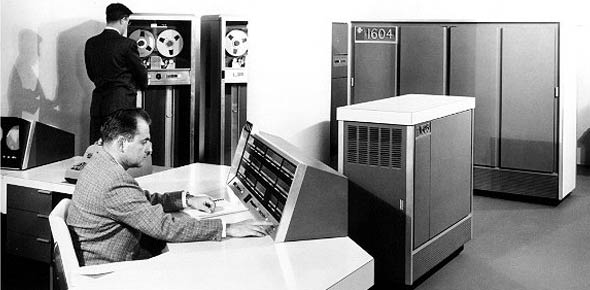# 2a553a CDC Volume 4

100 Questions | Attempts: 813
ShareSettingsVolume 4 of the Comm/Nav CDCs

• 1.
What is the purpose of VOR?
• A.

Determine bearing to a stations

• B.

Indicate distances along the approach

• C.

Determine left/right guidance

• D.

Determine up/down guidance

• 2.
The basic operating principle of VOR is phase comparing the
• A.

90 and 150 Hz modulated signals

• B.

30 Hz reference and 9,960 Hz FM signals

• C.

30 Hz variable and 9,960 Hz FM signals

• D.

30 Hz reference and 30 Hz variable signals

• 3.
If the magnetic bearing to a VOR station is 225 degrees, the phase difference between the 30 Hz reference and 30 Hz variable signal is
• A.

225 degrees

• B.

135 degrees

• C.

15 degrees

• D.

45 degrees

• 4.
VOR identification (audible) signals are transmitted
• A.

At 20 second intervals

• B.

At specified time intervals

• C.

At 30 second intervals

• D.

Continuously

• 5.
The ILS localizer course width at a distance of 10 miles from the transmitter is normally
• A.

0.7 degrees

• B.

2.5 degrees

• C.

0.5 degrees

• D.

5 degrees

• 6.
What frequency is used to transmit ILS glide slope information?
• A.

HF

• B.

VHF

• C.

LF

• D.

UHF

• 7.
The ILS marker beacon carrier frequency is
• A.

68.1 MHz

• B.

75 kHz

• C.

75 MHz

• D.

68.1 kHz

• 8.
What MLS outputs are provided to the pilot's and copilot's HSIs for approach and takeoff?
• A.

Ground station indications

• B.

Azimuth signals

• C.

Steering indications

• D.

Elevation signals

• 9.
What is not an MLS output?
• A.

Azimuth deviation

• B.

Azimuth warning flag

• C.

Glide slope correction flag

• D.

Glide slope deviation

• 10.
On how many channels are the MLS receivers capable of operating?
• A.

559

• B.

500

• C.

669

• D.

200

• 11.
What is the frequency range of the MLS receivers?
• A.

5031-5091 MHz

• B.

200-500 MHz

• C.

500-669 MHz

• D.

669-5031 MHz

• 12.
Which antennas are shared by MLS numbers 1 and 2?
• A.

3 and 4

• B.

3 and 2

• C.

1 and 3

• D.

1 and 2

• 13.
Which MLS landing system component allows for selection of system status?
• A.

• B.

Integrated display computer unit

• C.

Flight director mode selector

• D.

Data bus coupler

• 14.
When an ILS frequency is selected, the ARN-127 VOR receiver parks the HSI bearing pointer at the
• A.

10 o'clock position

• B.

6 o'clock position

• C.

3 o'clock position

• D.

4 o'clock position

• 15.
Which radial will the aircraft be positioned on if the aircraft is located north of the VOR station?
• A.

090 degrees

• B.

270 degrees

• C.

000 degrees

• D.

180 degrees

• 16.
To get a FROM flag in view on the HSI when the magnetic bearing to the VOR station is 75 degrees, the selected course should be manually set to
• A.

75 degrees

• B.

360 degrees

• C.

150 degrees

• D.

210 degrees

• 17.
During ILS operation, a full-scale deflection of the HSI vertical needle indicates the aircraft is off course from the runway centerline by
• A.

2.5 degrees

• B.

200 feet

• C.

400 feet

• D.

0.6 degrees

• 18.
In ILS operation, a deflection of two dots on the attitude director indicator glide slope deviation scale indicates the aircraft is
• A.

2.5 degrees off glide path

• B.

6.0 degrees off glide path

• C.

0.6 degrees off glide path

• D.

0.25 degrees off glide path

• 19.
When the ILS marker beacon amber (orange) lamp illuminates, the pilot hears a
• A.

3,000 Hz tone

• B.

1,000 Hz tone

• C.

1,300 Hz tone

• D.

400 Hz tone

• 20.
The total number of VOR/ILS channels that can be selected by the AN/ARN-147 control box is
• A.

240

• B.

200

• C.

40

• D.

160

• 21.
If the VOR station signal is lost or the receiver malfunctions, the ARN-147 system parks the bearing pointer at the
• A.

12 o'clock position

• B.

3 o'clock position

• C.

9 o'clock position

• D.

6 o'clock position

• 22.
In VOR mode, what information is sent to the HSI by the ARN-147 VOR/localizer receiver?
• A.

• B.

Left/right deviation, flag alarm, and TO/FROM

• C.

Relative bearing, flag alarm, and heading

• D.

Flag alarm, distance, and left/right deviation

• 23.
What glide slope information is provided to the attitude director indicator when the AN/ARN-127 is oeprating in the ILS mode?
• A.

• B.

Audio signals

• C.

Left/right deviations

• D.

Up/down deviations

• 24.
The main reference burst is transmitted when the main lobe of the TACAN surface beacon radiation pattern is due
• A.

South

• B.

North

• C.

West

• D.

East

• 25.
A TACAN beacon provides a station identification code every
• A.

7.5 seconds

• B.

20 seconds

• C.

40 seconds

• D.

30 seconds

## Related TopicsBack to top
×

Wait!
Here's an interesting quiz for you.